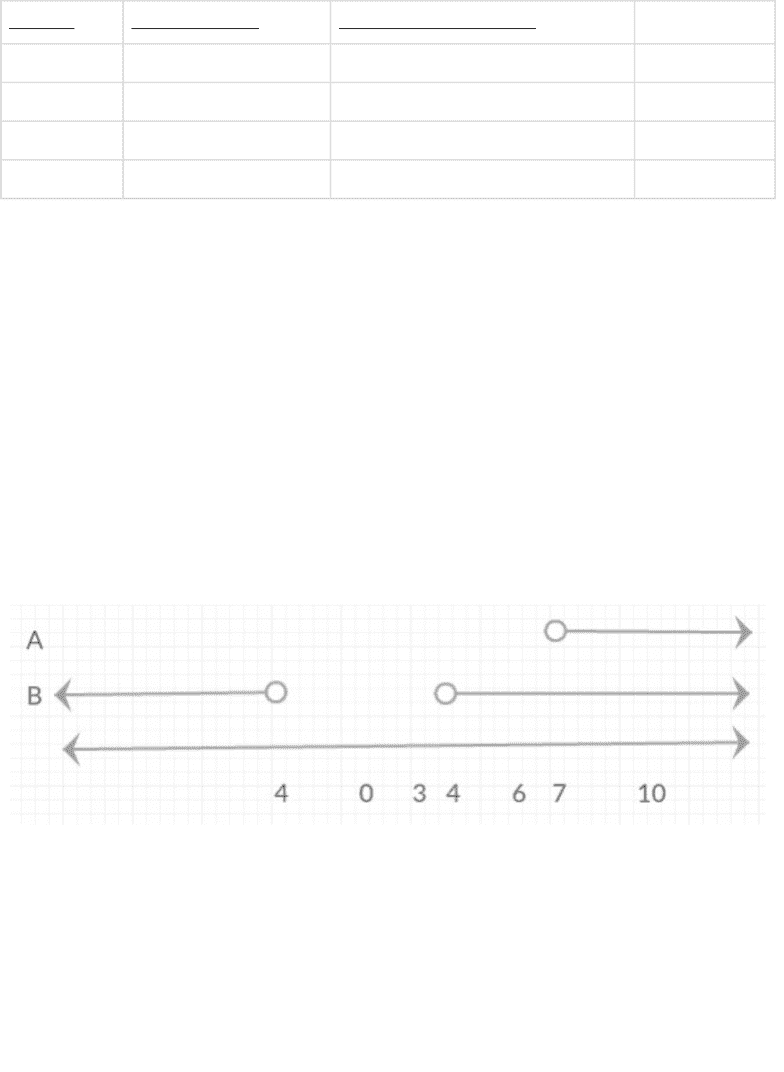Class Notes (1,000,000)
CA (620,000)
UTSG (50,000)
CSC (1,000)
CSC165H1 (200)
Lecture 2

CSC165H1 Lecture Notes - Lecture 2: Venn Diagram, Counterexample

Department
Computer Science
Course Code
CSC165H1
Professor
Ilir Dema
Lecture
2

This preview shows pages 1-2. to view the full 6 pages of the document.CSC165H1 – Lecture 2 – Universally Quantified Implication
A universal statement says that a certain property is true for all elements in a set. For
example,
"All positive numbers are greater than zero." (The set mentioned here is all positive numbers.)
A conditional/implication statement says that if one thing is true (this is the condition), then
another thing has to be true (this is the implication).
For example: If 378 is divisible by 18, then 378 is divisible by 6. The condition here is that 378 is
divisible by 18, and the implication is that 378 is also divisible by 6.
Boolean statements are statements that can be either true or false. Boolean claims are a
frequent logical form for claims.
Here is such a claim: "Every (real) number larger than seven has the property such that its
square is larger than sixteen."
We'll name this claim C0.
Notice that C0 is a complete English sentence. C0 claims something about all real numbers
greater than 7.
Since 165 > 7, C0 claims that the square of 165 is larger than sixteen.
Since 10 > 7, C0 claims that the square of 10 is larger than sixteen.
Since 7.5 > 7, C0 claims that the square of 7.5 is larger than sixteen.
However,
Six is NOT larger than seven: C0 does not directly claim anything about six. Therefore,
although the square of six is larger than sixteen, that is irrelevant to determining
whether C0 is true. The initial condition is not met.
Three is not larger than seven. C0 does not directly make a claim about three. Although
the square of three is less than sixteen (the second part of the statement is true), the
initial condition of the statement is not met, therefore it is irrelevant to determining
whether C0 is true.
Negative one hundred and sixty five is not larger than seven. Again, C0 does not directly
claim anything about -165. Therefore, it is also irrelevant to determining whether C0
is true.
All of these examples are called irrelevant cases- they are irrelevant to determining whether
C0 is true.
We can illustrate our results in several ways. First, we create a table:

Only pages 1-2 are available for preview. Some parts have been intentionally blurred.Number Greater than 7? Square greater than 16?
10 True True Okay
6 False True Irrelevant
3 False False Irrelevant
-165 False True Irrelevant
In general, these are the three cases that can arise in a conditional/implication statement:
The condition is true and the implication is true,
The condition is false and the implication is true (this is irrelevant) or
The condition is false and the implication is false (also irrelevant).
If the condition is true and the implication is false, that means the whole claim is disproved,
therefore we do not mention this case.
More simply,
T -> T Okay
F -> T Irrelevant
F -> F Irrelevant
The results of C0 can also be represented with a number line. We consider two sets of
numbers: A- the set of numbers larger than seven, and B- the set of all numbers that when
squared, are larger than sixteen. On a number line, these look like this:
For C to be true, A has to be completely contained in B. We can see from the number line that
every element of A is an element of B, but not every element of B is an element of A. Therefore
A is contained in B. (In other words, A is a subset of B.) This gives us the idea that C is true,
because it means that all elements in A have the properties of B. Note: this is not a proof.
A third option for representing the results is a Venn diagram. A Venn diagram may be helpful in
illustrating relationships between sets of things that are not numbers.
The enclosing rectangle of a Venn diagram represents the universe, the type of the things being
discussed. For C this is the set of real numbers,. The things in a set are called its elements or
members.